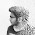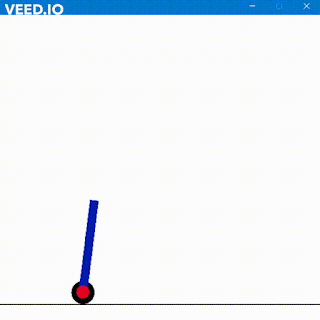### Convolutional Neural Networks IV

In this post, I will deal with back propagation, gradient descent etc. Do check out my previous posts regarding Max Pooling, filters, dropout layers, fully connected layers and CNN. To begin click here.
So

## What is Back Propagation?

Backpropagation is done whenever we find some error. After obtaining the probabilities of the images the CNN subtracts the actual answer with the obtained answer. Clearly, this is an error. So our first objective is too reduce this error. While I was working with the probabilities with the image of the letter 'R' (click here to know about the image 'R'), I got the probability of R as 0.911 and the probability of some other is 0.42.

Actual probability of getting a 'R' is 1 thus, the error is |1 - 0.911| = 0.081
The actual probability of not getting a 'R' is 0 thus, the error is   |0 - 0.42| = 0.42.
Thus the total error is 0.501. Now comes the power of weights. These weights are used in the gradient descent optimization algorithm which is used to find the minimum of the function. These weights are kept on adjusting along the gradient descent and each and every time the slope is chosen which produces the minimum error (like here we have 0.501). This slope is then fed in the equation which is evaluated to find the weights and that weight is multiplied with the input coming from every feature of the neuron (values) and then added with the bias to get the final value. This whole operation is performed several times to update the weights with appropriate values and updated within the network.

Consider the following image below
The red dot moves across the graph and tries to find the minimum error with the weight axis. This is performed for every neuron pair and their weights are then noted down. After finding the appropriate weights for every neuron pair, these weights are updated with their corresponding neuron pair and the process of NN is carried out again and again.

This gradient function is also known as the cost function if you are aware of that. Our main objective for that cost function is to minimize. In gradient descent, we jump or take steps towards negative side i.e downwards. The opposite is the case of gradient ascent where we jump towards up to find the maximum. Well, the good thing is that these objectives are executed by the NN itself automatically to gather the best parameter but there are also various parameters where it cannot be automatically done. These include the number of hidden layers, choosing the activation function, the right amount of stride, choosing the matrix size of the pooling layer, number of epochs, batch size and various other parameters.

All these can be achieved by trial and error as I do the same way to find my best NN. Although this hurts because to run a NN my Core i3 CPU (3rd Gen 😑) it takes around 3 hours to find the appropriate parameters. Well, hard works do pay off so keep on trying and explore the world of NN.

Cheers 😃

1.nice.do u have CNN handwritten in verilog

2.Well Not in handwritten though. I have tried simple neural networks for implementation of Logic gates in Verilog not CNN. Currently learning image processing to imply CNN.

Thank YOu

3.oh that good.can you please send your code to my mail sunil.bhukya424@gmail.com

1.I'll have to recheck it once for its working condition as I made it last year. I'll send the code as soon as possible.

Thanks

4.5.Hi, sir, I have three questions in CNN (I) to ask you.

Question 1:
The two side-by-side white-red images are identical and they are no different.

Question 2:
The two side-by-side white-gray images, the value of each cell in the right image is greater than the value of each cell in the left image.
How did this happen? The derivation step seems to be omitted.

Question 3:
A white-blue image is obtained by applying three filters. How do you get this white-blue image ?
What is the content of these three filters? thank you very much.

6.can you send me the code OF NN and CNN in verilog